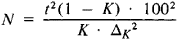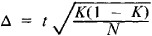# Instantaneous Observations, Method of

The following article is from The Great Soviet Encyclopedia (1979). It might be outdated or ideologically biased.

## Instantaneous Observations, Method of

in statistics, the fixing of the different states of a process being studied at definite moments of time, neglecting the duration of each of these states. The method is a variant of the sampling survey and is used, for example, for studying the distribution of work time and the operation of production equipment in industry, consumer demand in retail trade, and the use of rolling stock in railroad transport. It also allows us to obtain necessary information for calculating the fundamental characteristics of queuing processes (flow of requests per unit time and mean level of servicing). The method is most applicable for determining the efficiency of variations in the organization of the work of office employees, engineers, and technologists.

The method of instantaneous observations consists in carrying out observations over random or constant intervals of time with records on the state of the object being investigated at various moments of time. Special investigators check the work stations along a prescribed route during a work shift and determine the elements of work or machine time (“work” or “idle time”). The number of observations is calculated using the formulawhere K is the time utilization factor; Δk is the required precision, that is, the relative maximum error in determining K with confidence coefficient 0.954 or 0.997; and t is the guaranteed precision factor of the results of an instantaneous observation. Under stable production process conditions, t = 2, so that the confidence coefficient Φ = 0.954; under unstable production process conditions, t = 3 and Φ(t)= 0.997. The number of circuits is determined by dividing the number of entries by the number of work stations or by the number of installed pieces of equipment. The results of an instantaneous observation are estimated by the formulawhere (1 − K) represents the losses of time.

### REFERENCES

Barnes, P. “Vyborochnoe izuchenie rabochego vremeni sposobom mgnovennykh nabliudenii.” In Primenenie statisticheskikh metodov v proizvodstve. Moscow, 1963.
Onoprienko, G. K. Vyborochnyi analiz ispol’zovaniia rabochego vremeni. Moscow, 1968.
Il’enkova, S. D. Rezervy proizvodstva. Moscow, 1973.

A. G. SHIFMAN